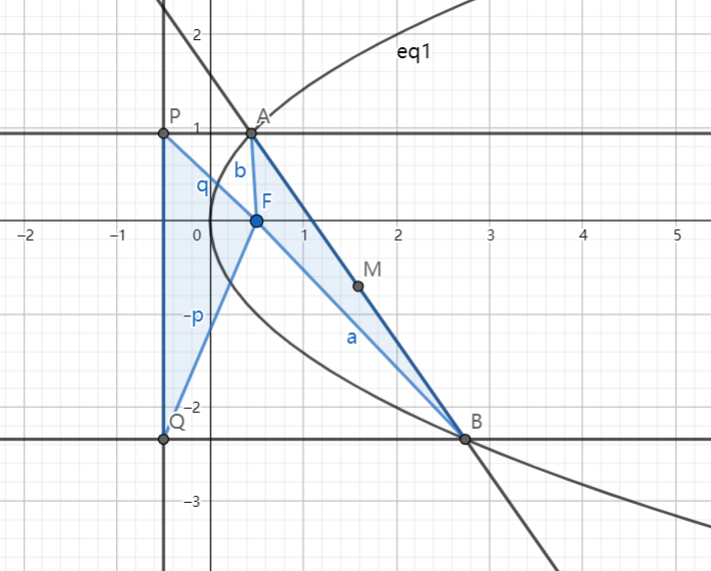# SAT1000 - P787

Geometry Level pendingAs shown above, the focus of the parabola $C: y^2=2x$ is $F$. Two lines $l_1, l_2$ which are parallel to the x-axis intersect with $C$ at $A,B$ and intersect with the directix at $P,Q$. $M$ is the midpoint of $AB$.

If $S_{\triangle PQF}=2S_{\triangle ABF}$, find the locus of point $M$.

If the locus can be expressed as $f(x,y)=0$, then when $y=50$, submit the sum of all possible value(s) for $x$.

Note: $S_{\triangle ABC}$ denotes the area of $\triangle ABC$.

Have a look at my problem set: SAT 1000 problems

×